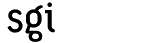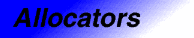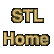# uninitialized_copy_nCategories: allocators, algorithms Component type: function

### Prototype

```template <class InputIterator, class Size, class ForwardIterator>
ForwardIterator uninitialized_copy_n(InputIterator first, Size count,
ForwardIterator result);
```

### Description

In C++, the operator new allocates memory for an object and then creates an object at that location by calling a constructor. Occasionally, however, it is useful to separate those two operations.  If each iterator in the range [result, result + n) points to uninitialized memory, then uninitialized_copy_n creates a copy of [first, first + n) in that range. That is, for each iterator i in the input range, uninitialized_copy_n creates a copy of *i in the location pointed to by the corresponding iterator in the output range by calling construct(&*(result + (i - first)), *i).

### Definition

Defined in the standard header memory, and in the nonstandard backward-compatibility header algo.h. This function is an SGI extension; it is not part of the C++ standard.

### Requirements on types

• InputIterator is a model of Input Iterator.
• Size is an integral type.
• ForwardIterator is a model of Forward Iterator.
• ForwardIterator is mutable.
• ForwardIterator's value type has a constructor that takes a single argument whose type is InputIterator's value type.

### Preconditions

• n >= 0
• [first, first + n) is a valid range.
• [result, result + n) is a valid range.
• Each iterator in [result, result + n) points to a region of uninitialized memory that is large enough to store a value of ForwardIterator's value type.

### Complexity

Linear. Exactly n constructor calls.

### Example

```class Int {
public:
Int(int x) : val(x) {}
int get() { return val; }
private:
int val;
};

int main()
{
int A1[] = {1, 2, 3, 4, 5, 6, 7};
const int N = sizeof(A1) / sizeof(int);

Int* A2 = (Int*) malloc(N * sizeof(Int));
uninitialized_copy_n(A1, N, A2);
}
```

### Notes

 In particular, this sort of low-level memory management is used in the implementation of some container classes.

 Uninitialized_copy_n is almost, but not quite, redundant. If first is an input iterator, as opposed to a forward iterator, then the uninitialized_copy_n operation can't be expressed in terms of uninitialized_copy.